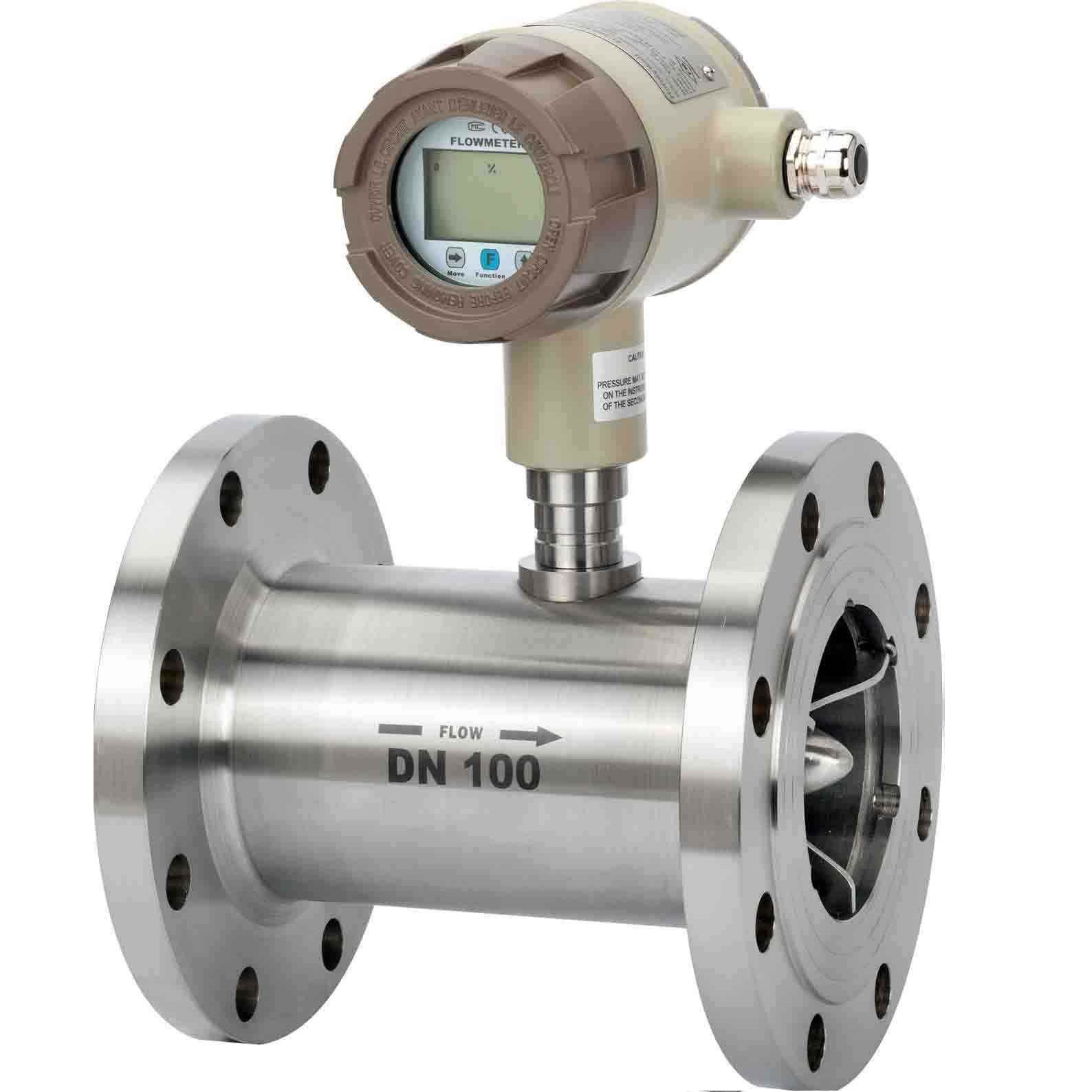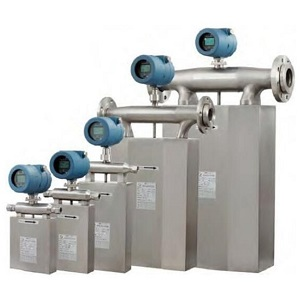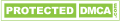# Flow meter volumetric and mass flow rate?

## Main difference

Some flow meters measure mass flow, some flow meter measure gas or air volumetric flow, what is the difference? Mass flow can be applied to calculate the total number of molecules of a gas  or air that is flowing. Volumetric flow calculates the amount of space occupied by air or gas molecules. The key difference being that volumetric flows can vary as gases can be compressed; the rates can fluctuate due to changes in temperature and pressures.

## How volumetric flow measured?

Measuring volumetric flow is done by calculating the 3-dimensional space occupied by the gas, while under controlled temperature and pressure. Also called the actual flow rate, turbine flow meter, vortex flow meters are normal used volumetric gas or air flow meter.How mass flow measured?

Measuring mass flow is done by calculating the number of molecules that pass through the flow measurement instrument. , the amount of space they occupy is not relevant. It is common to express mass flow rates as a normalised volumetric flow rate; in other words, measuring the amount of space these molecules would occupy under standard pressure(STP) and temperature conditions. (NTP). Gas or air mass flow rate measured is often released by Coriolis flow meter or thermal mass flow meter.Users should know first what they want to measure, and then choose gas volumetric flow meter or mass flow meter, but which measure you choose depends on your objectives.

Ideal Gas Law

This describes the correlation between temperature, volume, mass and pressure for static gases.

• PV=nRT
• Us such;
• P = Static pressure
• V = Volume
• n = Molar mass
• R = Gas constant
• T = Absolute temperature'

If we take all other factors as constant. If gas pressure (P) is increased for a factor of 2, the volume of this gas (V) will decrease for a factor of 2. This a common occurrence due to the compressibility of gases, as their molecules are pushed together due to an increase in pressure, their volume will decrease, the opposite happens when pressures are decreased. Although the volume may increase or decrease the number of molecules remains static.

## How the ideal gas law is impacted by flow rates

The actual space that air can take up when set in motion as gas flows vary in the same fashion as static air depending on pressure levels. If you double the pressure in the line you half the (VFR) Volumetric Flow Rate; the opposite is the same. The critical difference is that the number of molecules(mass flow rate) remains the same

## Which flow rate to choose and why?

When to use mass flow rate vs volumetric flow rate

Some gas flow meters provide accurate readings for both volumetric flow and mass flow. They can be utilized to measure the flow needed for your applications. Other flow controllers are designed to facilitate a closed-loop control to measure wither flow depending on your need.

When you want to focus on flow control or to measure the gas choose the mass flow option. When you need to monitor the number of molecules, mass flow measurement is the answer. If you are operating gas mixing apps, it is best to measure mass flow rates; this ensures that the constituent gas remains in the correct concentration levels. You can utilise Custody transfer applications if the space occupied by the gas is of less importance than the mass of the gas being used.

If the focus of the control or flow measurement is what is within the gas volume, choose to measure volumetric flow. When you are monitoring the gas streams components under real-time process conditions, you should use volumetric flow measurements. This is also best used when monitoring ambient air or industrial hygiene applications, especially when the overall goal is to determine the number of particles in a volume of air when measuring under actual conditions.